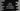# Java program to find the maximum element in a HashSet## How to find the maximum element in a HashSet in Java:

HashSet class implements the Set interface of Java. To get the maximum element in a HashSet, we can either run a loop and find out the maximum or we can use the predefined method max defined in Collections.

In this post, I will show you how to do this in both of these ways.

## Method 1: By using a loop:

First of all, let’s try to find the largest value in a HashSet using a loop. We will use one for loop to iterate through the elements of the HashSet. The below algorithm will be used :

### Algorithm:

• Create one variable to hold the largest value. Initialize it with Integer.MIN_VALUE, which is the minimum value for an integer.
• Run a for loop and iterate through the numbers in the hashset. For each value found while iterating, compare it with the largest value holder variable. If it is more than that, assign this value as the largest value. Else, move to the next element.

HashSet doesn’t guarantee the iteration order. If we run a for loop, each time it might iterate differently. But, finally, it will give us the largest value.

• Once the loop ends, print out the largest value holder variable, which is the largest value in the HashSet.

### Java program to find the largest element in a HashSet by using a for loop:

Below is the complete Java program:

``````import java.util.HashSet;

public class Main {
public static void main(String[] args) {
int MAX = Integer.MIN_VALUE;

HashSet<Integer> hashSet = new HashSet<Integer>() {{
}};

for (int item : hashSet) {
if (item > MAX) {
MAX = item;
}
}

System.out.println("Max value in the given HashSet : " + MAX);
}
}``````

This program is using the same steps that we discussed above.

Here,

• MAX is initialized as the minimum value of Integer. This variable is used to hold the maximum value in the hashset.
• hashSet is the given HashSet. We have added 6 numbers to this HashSet.
• To iterate through the elements of this HashSet, we are using a for in loop. This loop iterates through the values of hashSet. For each value, it compares it with MAX. If it is greater than MAX, then it assigns this value to MAX.
• Finally, after the loop ends, we are printing the value of MAX, which is the largest value in the hashset.

It will print the below output:

``Max value in the given HashSet : 60``

## Method 2: By using Collections.max():

Collections.max method can be used to find the maximum value in a HashSet. This method takes one collection as its parameter and returns the maximum value in that collection. Each element in the collection should be mutually comparable. If we have any custom objects in the HashSet, then we need to make sure that it implements the Comparable interface so that two objects can be compared.

It returns the maximum value in the collection as per the natural ordering of the elements.

For an empty collection, it will throw NoSuchElementException and if the elements are not comparable, then it will throw ClassCastException.

Internally, it uses an iterator to iterate through the elements of the collection and finds out the max.

We can use this method in the above program to find the largest value in the HashSet.

### Java program to find the maximum HashSet value by using Collections.max():

Below is the complete Java program:

``````import java.util.Collections;
import java.util.HashSet;

public class Main {
public static void main(String[] args) {

HashSet<Integer> hashSet = new HashSet<Integer>() {{